#### You may also like### Telescoping Series

Find $S_r = 1^r + 2^r + 3^r + ... + n^r$ where r is any fixed positive integer in terms of $S_1, S_2, ... S_{r-1}$.### Degree Ceremony

What does Pythagoras' Theorem tell you about these angles: 90°, (45+x)° and (45-x)° in a triangle?### OK! Now Prove It

Make a conjecture about the sum of the squares of the odd positive integers. Can you prove it?

# Vanishing Point

##### Age 14 to 18 Challenge Level:
You may wish to take a look at Diminishing Returns before working on this problem.

In the interactive environment below, you can move the slider to see how the image is built up.

At each stage of the process, what proportion of the image is coloured blue?

What would happen if we continued the process forever?

Once you've had a chance to think about these questions, click below to see some different approaches to understanding the process.

Here are the first three stages of the process: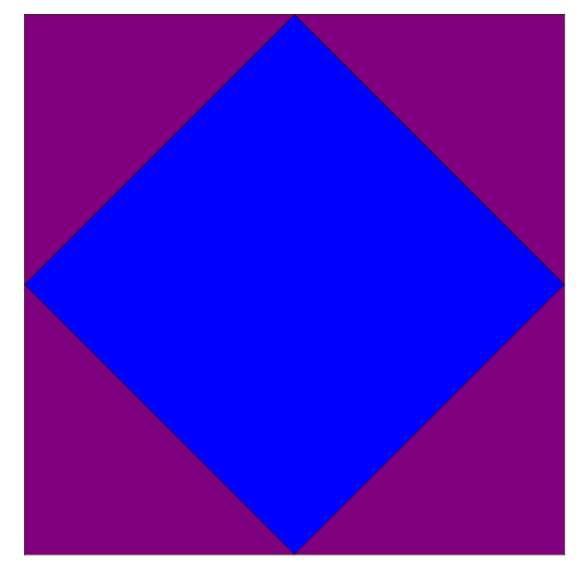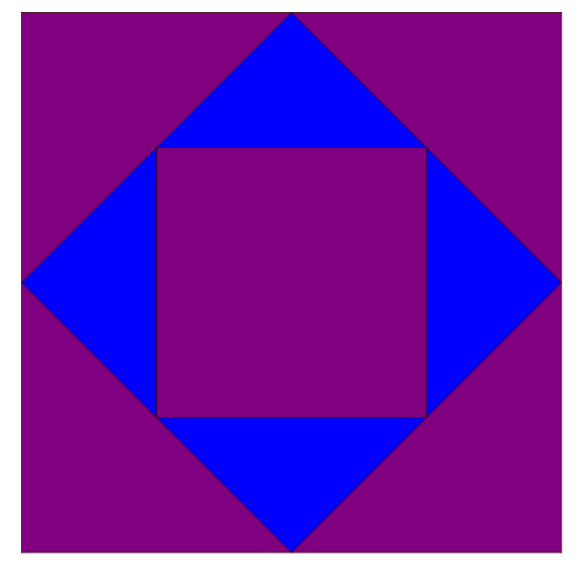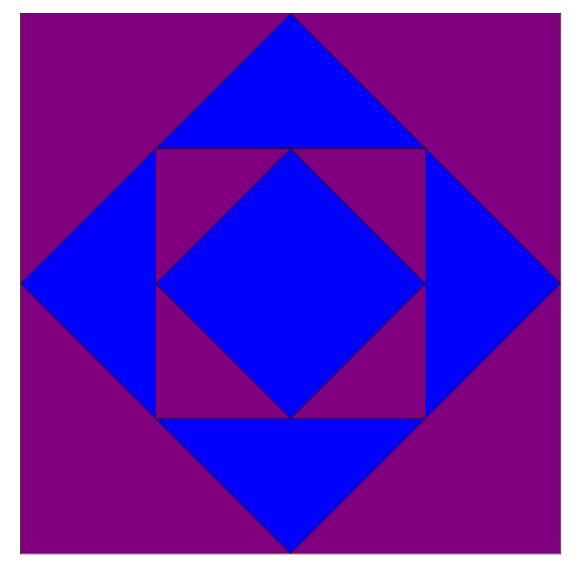In the first picture, the blue area is $\frac12$.
In the second picture, the blue has been partly covered by a purple square with area $\frac14$ so the blue area is $\frac12-\frac14$.
In the third picture, a blue square with area $\frac18$ has been added. As the pattern continues, the blue area will be $\frac12-\frac14+\frac18-\frac{1}{16}+\frac{1}{32}-...$

Instead of looking at how the pattern builds up, we could divide the completed pattern into the nested sections:This shows the outer ring of the pattern, in which there are four larger purple triangles and four smaller blue triangles with half the area. So the ring is made up of twice as much purple as blue.

The same will be true for each ring added inside, therefore the overall pattern has twice as much purple as blue.

Here's another way to look at the completed pattern: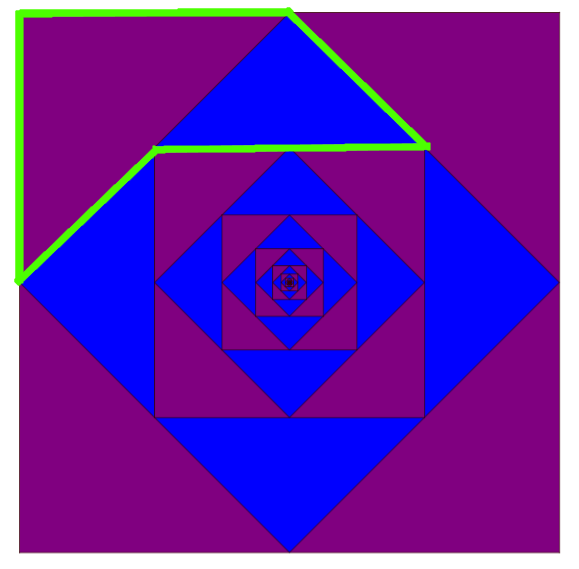$\frac23$ of the highlighted shape's area is purple, and $\frac13$ is blue.

The whole pattern can be subdivided into pieces like this, so that means $\frac23$ of the total area of the pattern must be purple, and $\frac13$ of the total area must be blue.

Below is a collection of images created using repeating processes.

For each pattern, can you find a sequence of fractions whose sum represents the blue area?
For each pattern, can you find different ways to work out the total proportion shaded blue?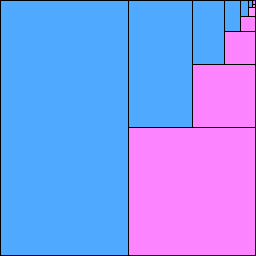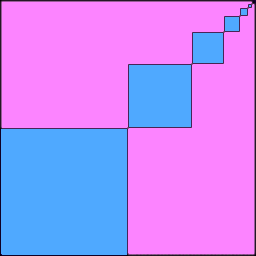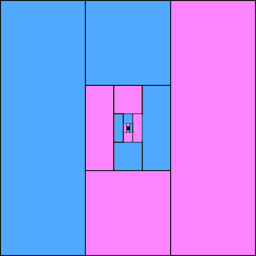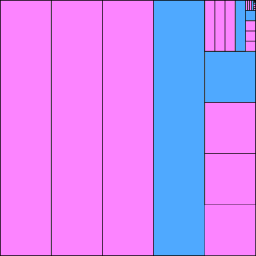Can you come up with your own pictures to represent the sums of other series?